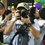# One minus one plus one minus one plus...

There are three types of people in this world:

Evaluate:

$\color{#3D99F6}{S}=1-1+1-1+1-1+\ldots$

Type 1

$\color{#3D99F6}{S}=(1-1)+(1-1)+(1-1)+\ldots=0+0+0+\ldots=\boxed{0}$

Type 2

$\color{#3D99F6}{S}=1-(1-1)-(1-1)-(1-1)-\ldots=1-0-0-0-\ldots=\boxed{1}$

But the $\displaystyle 3^{rd}$ type of people did like this:

$1-\color{#3D99F6}{S}=1-(1-1+1-1+\ldots)=1-1+1-1+1-1+\ldots = S$

$\Leftrightarrow 1-\color{#3D99F6}S=\color{#3D99F6}S \Rightarrow 2\color{#3D99F6}S=1 \Rightarrow \color{#3D99F6}S=\boxed{\frac{1}{2}}$5 years, 11 months ago

This discussion board is a place to discuss our Daily Challenges and the math and science related to those challenges. Explanations are more than just a solution — they should explain the steps and thinking strategies that you used to obtain the solution. Comments should further the discussion of math and science.

When posting on Brilliant:

• Use the emojis to react to an explanation, whether you're congratulating a job well done , or just really confused .
• Ask specific questions about the challenge or the steps in somebody's explanation. Well-posed questions can add a lot to the discussion, but posting "I don't understand!" doesn't help anyone.
• Try to contribute something new to the discussion, whether it is an extension, generalization or other idea related to the challenge.

MarkdownAppears as
*italics* or _italics_ italics
**bold** or __bold__ bold
- bulleted- list
• bulleted
• list
1. numbered2. list
1. numbered
2. list
Note: you must add a full line of space before and after lists for them to show up correctly
paragraph 1paragraph 2

paragraph 1

paragraph 2

[example link](https://brilliant.org)example link
> This is a quote
This is a quote
    # I indented these lines
# 4 spaces, and now they show
# up as a code block.

print "hello world"
# I indented these lines
# 4 spaces, and now they show
# up as a code block.

print "hello world"
MathAppears as
Remember to wrap math in $$ ... $$ or $ ... $ to ensure proper formatting.
2 \times 3 $2 \times 3$
2^{34} $2^{34}$
a_{i-1} $a_{i-1}$
\frac{2}{3} $\frac{2}{3}$
\sqrt{2} $\sqrt{2}$
\sum_{i=1}^3 $\sum_{i=1}^3$
\sin \theta $\sin \theta$
\boxed{123} $\boxed{123}$

Sort by:

You forgot $4^\text{th}$ type of people; they say that this series diverges.

- 5 years, 10 months ago

Its answer oscillates b/w 0 and 1

- 5 years, 10 months ago

Yes that's why it diverges.

- 5 years, 10 months ago

Even more here:

Evaluate : $S=1-2+3-4+5-6+ \ldots$

Type 1 : $S=1+(-2+3)+(-4+5)+ \ldots = 1+1+1+1+\ldots=\infty$

Type 2 : $S=(1-2)+(3-4)+(5-6)+ \ldots = -1-1-1-1+\ldots=-\infty$

Type 3 : They go to WolframAlpha, search this:

 1 sum(n from 1 to infty,(-1)^n*n) 

Which shows up that "The ratio test is inconclusive." and "The root test is inconclusive.", from which they implies that the sum is incosistent.

Type 4 : They go to Wikipedia and finds out that the sum is actually equal to $1/4$.

- 5 years, 10 months ago

Wow! Awesome!

I also saw this video:

$1+2+3+4+\ldots=\frac{1}{12}$

- 5 years, 10 months ago

Wow, that's cool :)))

- 5 years, 10 months ago

i guess u forgot the negative sign along with 1/12

- 5 years, 10 months ago

last one is pretty good

- 5 years, 11 months ago

haha.. g8

- 5 years, 11 months ago

Grandi series.

- 5 years, 11 months ago

Gud 1

- 5 years, 10 months ago